# How to use the INDEX Excel functionThe INDEX function in Excel returns the value of a specified cell within an array. You essentially tell the function the array and the coordinates of the cell within the array, and the function returns the value of that cell. INDEX is useful on its own to pull data out of a larger dataset, or in combination with other functions like the MATCH function.

### Syntax

=INDEX(array, row_num, [column_num])
• array is the range of cells the function will reference
• row_num is the row number, within the array, of the cell to reference
• column_num is the column number of the cell to reference. If left blank, the function will default to column 1

### How to use the INDEX Excel function

In its most basic form, the INDEX function takes coordinates in the form of a row and column number, and uses those coordinates to find a cell within the specified array.

This is demonstrated in the example below.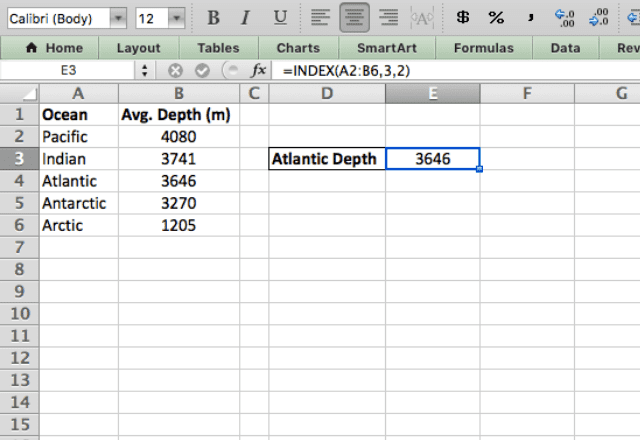Here I’ve used the formula =INDEX(A2:B6,3,2) to return the depth of the Atlantic Ocean. The function looks within the array A2:B6, and finds the cell in the third row and second column. As you can see, it properly returns the value in that cell!

It’s important to note that the INDEX function is a reference function, which means it doesn’t perform any calculation of its own.

Basically, all it does is locate a cell and reference the value within that cell. To demonstrate this, watch what happens when I change the value in the referenced cell.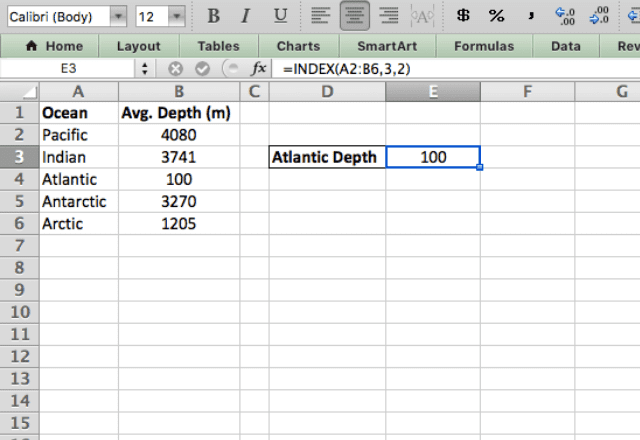I changed the Atlantic depth to 100, and the reference cell reflected the change. I didn’t touch the formula in E3, but it updated all on its own!

#### Reference form

The INDEX function is most commonly used in Array form, which is what I just showed. However, there’s an alternative form of this function called the Reference form.

In Reference form, the INDEX function can take multiple input arrays.

In order to do this, you have to specify which array you want to search. You can do this by adding a fourth parameter to the function:

=INDEX(reference, row_num, [column_num], [area_num])

The area_num parameter tells the function which reference array to look in. If left blank, it defaults to the first array.

To get a better idea of how INDEX works in Reference form, take a look at the next example.

I now have three tables of data. I can use the Reference form of the INDEX function to incorporate all three within a single function by typing =INDEX((A2:B6,D2:E6,G2:H6),5,2,2).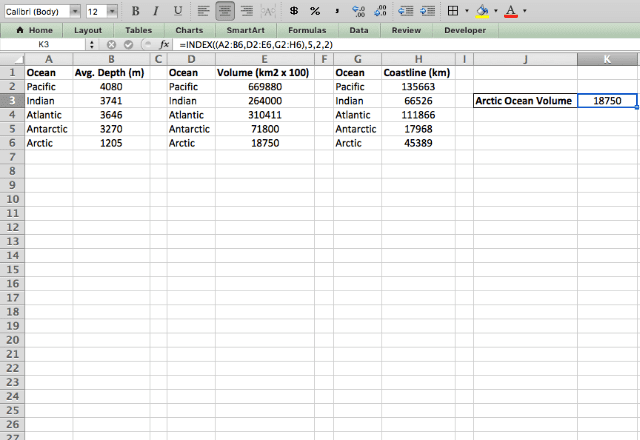As you can see from the formula, you can enter all three arrays for the reference parameter. Make sure you enclose them in brackets and separate them with commas.

Next, specify the row and column numbers to reference, just like in the earlier example.

Finally, there’s the area_num parameter at the end. This parameter (2) tells the function to look in the second array. After typing all this in, I get the correct result pulled from the data!

#### INDEX with COUNTA

As shown in the examples above, the INDEX function is very useful on its own. But like many functions, it really hits its stride when combined with other functions.

One useful example is combining INDEX with COUNTA.

This combination lets you automatically reference the last entry in a dataset, so that your INDEX function will always reference the most recent data. This is handy when you have a live spreadsheet that is updated regularly.

In the example below, I have some data on weekly rainfall. This table is updated every week, and I want to quickly have a reference to the latest numbers. I achieve this by typing =INDEX(A:B,(COUNTA(A:A)),2).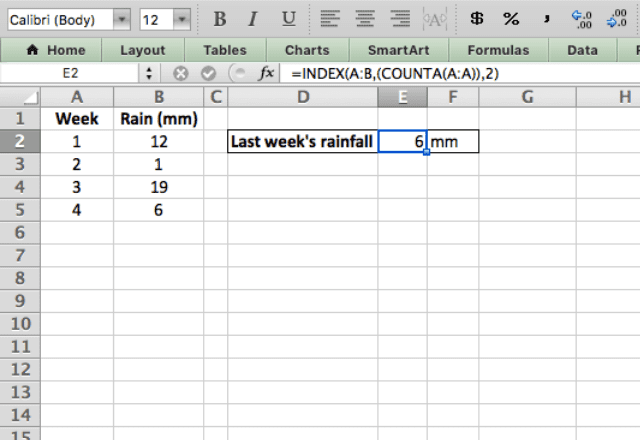Now how does this work? The function uses the entire A and B columns as its reference array, which means anything added to those columns will fall within the array.

To specify which row I want to reference, I use the COUNTA function to count how many rows are populated. If 5 rows are populated, then I want to reference row 5 – COUNTA makes this happen.

Now comes the real test: does the function automatically update when I add new data? In the screenshot below I’ve added week 5, and you can see that the function automatically changed to show this new data!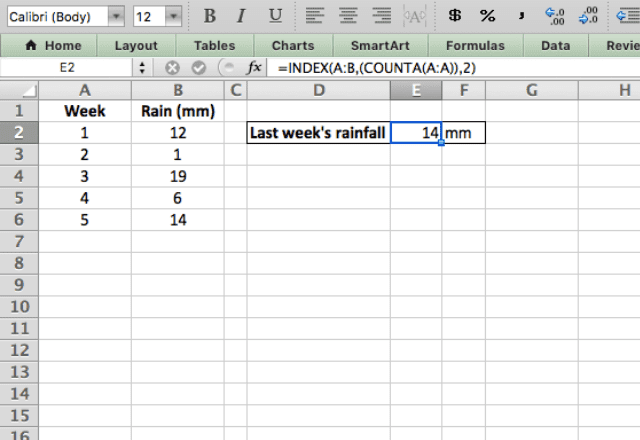### Final thoughts

Clearly, the INDEX function in Excel is quite powerful when combined with other functions.

Another common combination is INDEX and MATCH, which combines to form a more flexible version of the VLOOKUP function. I encourage you to check it out!